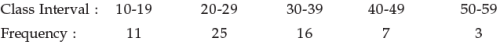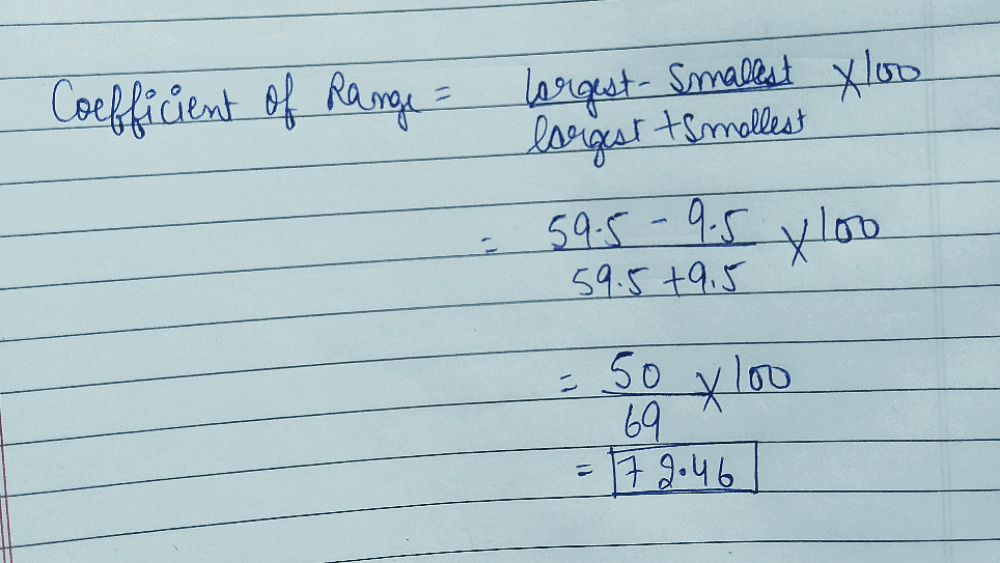What is the coefficient of range for the foll...

### Related TestWhat is the coefficient of range for the following distribution?• a)
22
• b)
50
• c)
72.46
• d)
75.82Ragul D
Class interval is regular so you have change the class interval into class boundaries
class interval frequency
9.5-19.5. 11
19.5-29.5. 25
29.5-39.5. 16
39.5-49.5. 7
49.5-59.5. 3
largest value=59.5
lowest value=9.5

coefficient of range = l-s/l+s ×100
= 59.5-9.5/59.5+9.5 ×100
=72.46

 1 Crore+ students have signed up on EduRev. Have you?Rakhi Jangra
What is the coefficient of range for the following distribution?a)22b)50c)72.46d)75.82Correct answer is option 'C'. Can you explain this answer?
Q3-Q1/Q3+ Q1*100
59-10/59+10*100= 72.46View courses related to this question Explore CA Foundation courses
 Explore CA Foundation coursesView courses related to this questionMobasshir Hassan
2Sneha Charaya
What is the coefficient of range for the following distribution?a)22b)50c)72.46d)75.82Correct answer is option 'C'. Can you explain this answer?Jaswant Patel
( c). L-S×100
L+S

Question Description
What is the coefficient of range for the following distribution?a)22b)50c)72.46d)75.82Correct answer is option 'C'. Can you explain this answer? for CA Foundation 2023 is part of CA Foundation preparation. The Question and answers have been prepared according to the CA Foundation exam syllabus. Information about What is the coefficient of range for the following distribution?a)22b)50c)72.46d)75.82Correct answer is option 'C'. Can you explain this answer? covers all topics & solutions for CA Foundation 2023 Exam. Find important definitions, questions, meanings, examples, exercises and tests below for What is the coefficient of range for the following distribution?a)22b)50c)72.46d)75.82Correct answer is option 'C'. Can you explain this answer?.
Solutions for What is the coefficient of range for the following distribution?a)22b)50c)72.46d)75.82Correct answer is option 'C'. Can you explain this answer? in English & in Hindi are available as part of our courses for CA Foundation. Download more important topics, notes, lectures and mock test series for CA Foundation Exam by signing up for free.
Here you can find the meaning of What is the coefficient of range for the following distribution?a)22b)50c)72.46d)75.82Correct answer is option 'C'. Can you explain this answer? defined & explained in the simplest way possible. Besides giving the explanation of What is the coefficient of range for the following distribution?a)22b)50c)72.46d)75.82Correct answer is option 'C'. Can you explain this answer?, a detailed solution for What is the coefficient of range for the following distribution?a)22b)50c)72.46d)75.82Correct answer is option 'C'. Can you explain this answer? has been provided alongside types of What is the coefficient of range for the following distribution?a)22b)50c)72.46d)75.82Correct answer is option 'C'. Can you explain this answer? theory, EduRev gives you an ample number of questions to practice What is the coefficient of range for the following distribution?a)22b)50c)72.46d)75.82Correct answer is option 'C'. Can you explain this answer? tests, examples and also practice CA Foundation tests.

• ### For a frequency distribution coefficient of skewness = 0.6; mean = 172 and ... more(Scan QR code)